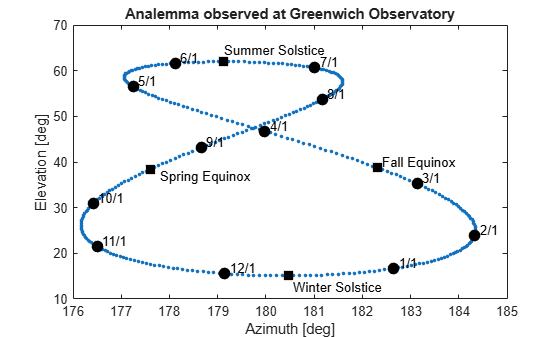# Estimate Sun Analemma Using Planetary Ephemerides and ECI to AER Transformation

This example shows how to estimate the analemma. The analemma is the curve that represents the variation of the angular offset of the Sun from its mean position on the celestial sphere relative to a specific geolocation on the Earth surface. In this example, the analemma is estimated relative to the Royal Observatory at Greenwich, United Kingdom. After the estimation, the example plots the analemma.

This example uses data that you can download using the aeroDataPackage function.

### Identify the Dates of a Year over Which to Calculate the Analemma of the Sun

Specify the dates for which to calculate the analemma. In this example, these dates range from January 1, 2014 to December 31, 2014 at noon UTC.

```dv = datetime(2014,1,1:365,12,0,0); dvUTC = [dv.Year' dv.Month' dv.Day' dv.Hour' dv.Minute' dv.Second'];```

### Calculate the Position of the Sun

Use the planetEphemeris function to calculate the position of the Sun. In this example:

• The tdbjuliandate function calculates the Julian date for the dynamic barycentric time (TDB).

• The tdbjuliandate function requires the terrestrial time (TT).

The calculation of the terrestrial time in seconds from UTC requires the difference in Coordinated Universal Time (UTC) and International Atomic Time (TAI).

• For 2014, this difference (dAT) is 35 seconds.

• The approximate terrestrial time (secTT) is the dAT + 32.184 seconds.

• The terrestrial time in year, month, day, hour, minutes, and seconds is contained in the dvTT array.

```dAT = 35; secTT = dAT + 32.184; dvTT = dv + secTT/86400;```

Estimate the Julian date for the dynamic barycentric time based on the terrestrial time using the dvTT array.

`jdTDB = tdbjuliandate([dvTT.Year' dvTT.Month' dvTT.Day' dvTT.Hour' dvTT.Minute' dvTT.Second']);`

Determine the position of the Sun for these dates:

`posSun = planetEphemeris(jdTDB,'Earth','Sun')*1000;`

### Calculate Difference Between UTC and Principal Universal Time (UT1)

Calculate the difference between UTC and UT1, deltaUT1, using the modified Julian dates for UTC.

```mjdUTC = mjuliandate(dvUTC); dUT1 = deltaUT1(mjdUTC);```

### Calculate Polar Motion and Displacement of the Celestial Intermediate Pole (CIP)

Calculate the polar motion and displacement of the CIP using the modified Julian dates for UTC.

```PM = polarMotion(mjdUTC); dCIP = deltaCIP(mjdUTC);```

### Specify the Geopotential Position of the Royal Observatory at Greenwich, United Kingdom

Specify the geopotential location for the position against which to estimate the analemma. In this example, this location is the latitude, longitude, and altitude for the Royal Observatory at Greenwich (51.48 degrees North, 0.0015 degrees West, 0 meters altitude).

```LLAGreenwich = [51.48,-0.0015,0]; aer = eci2aer(posSun,dvUTC,repmat(LLAGreenwich,length(jdTDB),1),... 'deltaAT',dAT*ones(length(jdTDB),1),'deltaUT1',dUT1,... 'PolarMotion',PM,'dCIP',dCIP);```

### Specify Days Within the Year of the Analemma That You Want to Plot

On the analemma, you can plot days of interest within the year of the analemma. This example plots:

• The first day of each month in 2014.

• The summer and winter solstices.

• The spring and fall equinoxes.

To get the first day of each month in 2014:

`aerFirstMonth = aer(dvUTC(:,3)==1,:);`

To get solstices and equinoxes (for 2014 are 3/20, 6/21, 9/22, 12/21):

```solsticeEquinox = [ aer(dvUTC(:,2)==3 & dvUTC(:,3)==20, 1:2); ... aer(dvUTC(:,2)==6 & dvUTC(:,3)==21, 1:2); ... aer(dvUTC(:,2)==9 & dvUTC(:,3)==22, 1:2); ... aer(dvUTC(:,2)==12 & dvUTC(:,3)==21, 1:2) ];```

### Plot Results

Plot the analemma. Along the analemma, plot points for the whole year, first days of the month, equinoxes, and solstices.

```firstDays = (12:-1:1) + "/" + 1; f = figure; plot(aer(:,1),aer(:,2),'.',... solsticeEquinox(:,1),solsticeEquinox(:,2),'ks',... aerFirstMonth(:,1),aerFirstMonth(:,2),'ko',... 'MarkerSize',8,'MarkerFaceColor','k'); title('Analemma observed at Greenwich Observatory'); xlabel('Azimuth [deg]'); ylabel('Elevation [deg]'); axis([176,185,10,70]) text(aerFirstMonth(:,1)+.1, aerFirstMonth(:,2)+1.2, firstDays, 'Color', 'k','HorizontalAlignment', 'Left'); text(solsticeEquinox(1,1)+.2, solsticeEquinox(1,2)-1.5, 'Spring Equinox', 'Color', 'k','HorizontalAlignment', 'Left'); text(solsticeEquinox(2,1), solsticeEquinox(2,2)+2.5, 'Summer Solstice', 'Color', 'k','HorizontalAlignment', 'Left'); text(solsticeEquinox(3,1)+.1, solsticeEquinox(3,2)+1.2, 'Fall Equinox', 'Color', 'k','HorizontalAlignment', 'Left'); text(solsticeEquinox(4,1)+.1, solsticeEquinox(4,2)-2.5, 'Winter Solstice', 'Color', 'k','HorizontalAlignment', 'Left');```